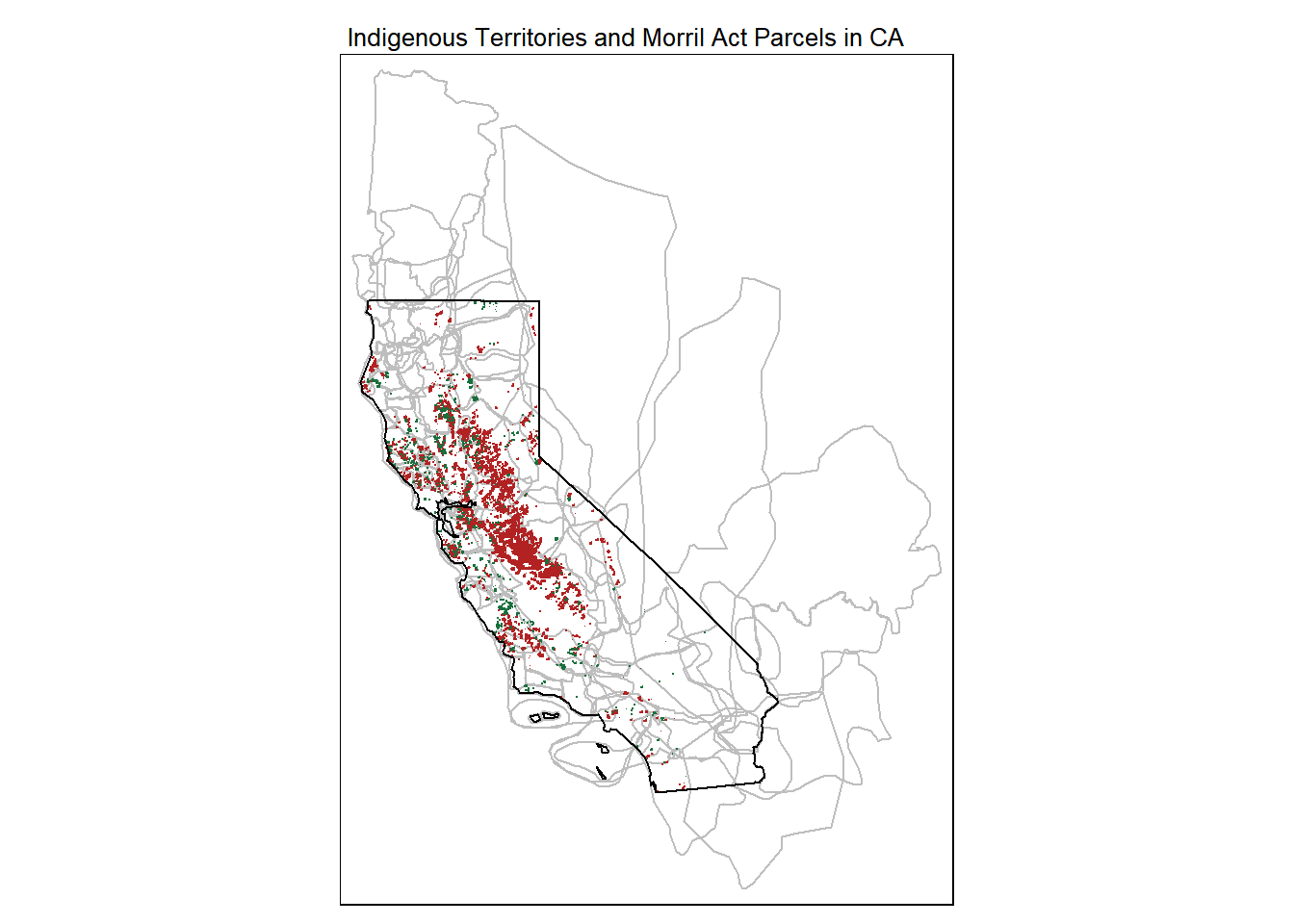DRAFT

# Summary

This notebook summarizes the ~150,000 acres of land that was transferred from the Federal Government to the State of California under the 1862 Morrill Act to support the establishment of the University of California. The distribution and acreage of land is broken down by the Native American tribal groups from whom the land had be seized.

Data sources and references:

``````library(dplyr)
library(tmap)
library(DT)
library(leaflet)
library(sf)
library(lubridate)
library(leaflet.providers)
library(leaflet.extras)``````

# 2. Import the data

Load the Morrill Act parcels for California:

``````data_dir <- "./data"

## Import the data and delete duplicate rows (same geometry + attributes)
hcnparc_ca_sf <- sf::st_read(file.path(data_dir, "hcnparc_loc_ca.geojson"), quiet = TRUE) %>%
distinct(across())``````

Load the indigenous territories that intersect California (data source):

``````territories_ca_fn <- file.path(data_dir, "territories_ca.geojson")

## Import the polygons and transform the to Lat-Long NAD83 (to match the parcels)
territories_ca_sf <- sf::st_read(territories_ca_fn, quiet = TRUE) %>%
st_transform(4269)``````

Double-check the data by mapping the parcels and territories together:

``````cabnd_sf <- sf::st_read(file.path(data_dir, "ca_bnd.geojson"), quiet = TRUE)

tm_shape(territories_ca_sf) +
tm_borders(col = "gray") +
tm_shape(hcnparc_ca_sf) +
tm_polygons(col = "#B22222", border.col = "#B22222") +
tm_shape(hcnparc_ca_sf %>% filter(LG_State == "CA")) +
tm_polygons(col = "#196F3D", border.col = "#196F3D") +
tm_shape(cabnd_sf) +
tm_borders(col = "black", lwd = 1) +
tm_layout(main.title = "Indigenous Territories and Morril Act Parcels in CA",
main.title.size = 0.8)``````# 3. Tabulate Parcels by Tribe

Here we summarize the number and acreage of parcels that were claimed by agents of the State of California to establish UC according to the original occupants. Note that due to overlap between Indigenous Territories, some parcels are double-counted in this table.

``````## Pull out parcels whose sale went to the State of CA, adding a unique id for the join
uc_prcl_sf <- hcnparc_ca_sf %>%
filter(LG_State == "CA") %>%
mutate(unique_id = 1:n())

## Do a spatial join
uc_prcl_ge_sf <- uc_prcl_sf %>%   ## 4269
st_join(territories_ca_sf)

## Compute the number and acreage of parcels for each Tribe
tribal_summary_tbl <- uc_prcl_ge_sf %>%
st_drop_geometry() %>%
group_by(Name) %>%
summarise(num_parcels = n(), acreage = sum(Acres), .groups = "drop_last")

DT::datatable(tribal_summary_tbl %>%
filter(!is.na(Name)),
class = 'row-border stripe hover compact',
colnames = c("Tribe", "Number Parcels", "Acreage"),
width = 500,
rownames = T,
options = list(autoWidth = TRUE,
paging = FALSE,
searching = FALSE,
info = FALSE)) %>%
formatRound(columns = "acreage", digits = 0) ``````

# 4. Explore the Map

The map below shows the parcels of public (federal) land claimed by the State of California under the Morrill Act to support the establishment of UC. Click on a parcel to view more info about it.

``````## Construct the names of the tribes for each parcel
uc_prcl_tribes_tbl <- uc_prcl_ge_sf %>%
st_drop_geometry() %>%
select(unique_id, Name) %>%
group_by(unique_id) %>%
summarise(tribes = paste(Name, collapse = "; "), .groups = "drop_last")

## Add the Tribes column to uc_prcl_sf
uc_prcl_tribes_sf <- uc_prcl_sf %>%
left_join(uc_prcl_tribes_tbl, by = "unique_id")

## Prepare a layer for leaflet
uc_prcl_ge_pdt_sf <- uc_prcl_tribes_sf %>%
st_transform(4326) %>%
st_zm() %>%
mutate(dp_year = as.integer(substr(Date_Patent,1,4)),
dp_month = as.integer(substr(Date_Patent,5,6)),
dp_day = as.integer(substr(Date_Patent,7,8))) %>%
mutate(dp_dt = make_date(dp_year, dp_month, dp_day)) %>%
mutate(prcl_popup = paste0("<p><b>Patentee:</b> ", Patentees, "</p>",
"<p><b>Beneficiary:</b> ", University, "<br/>",
"<b>Date:</b> ", format(dp_dt, "%d %B, %Y"), "<br/>",
"<b>Acres:</b> ", round(Acres, 1), "<br/>",
"<b>Land Desc:</b> ", MTRSA_LG, "<br/>",
"<b>Tribal territory(s):</b> ", tribes, "<br/>",
"<b>Source document:</b> <a href='", Parcel_Link, "'>GLO</a></p>")) %>%
select(prcl_popup)

## Create the leaflet object
m <- leaflet(uc_prcl_ge_pdt_sf) %>%